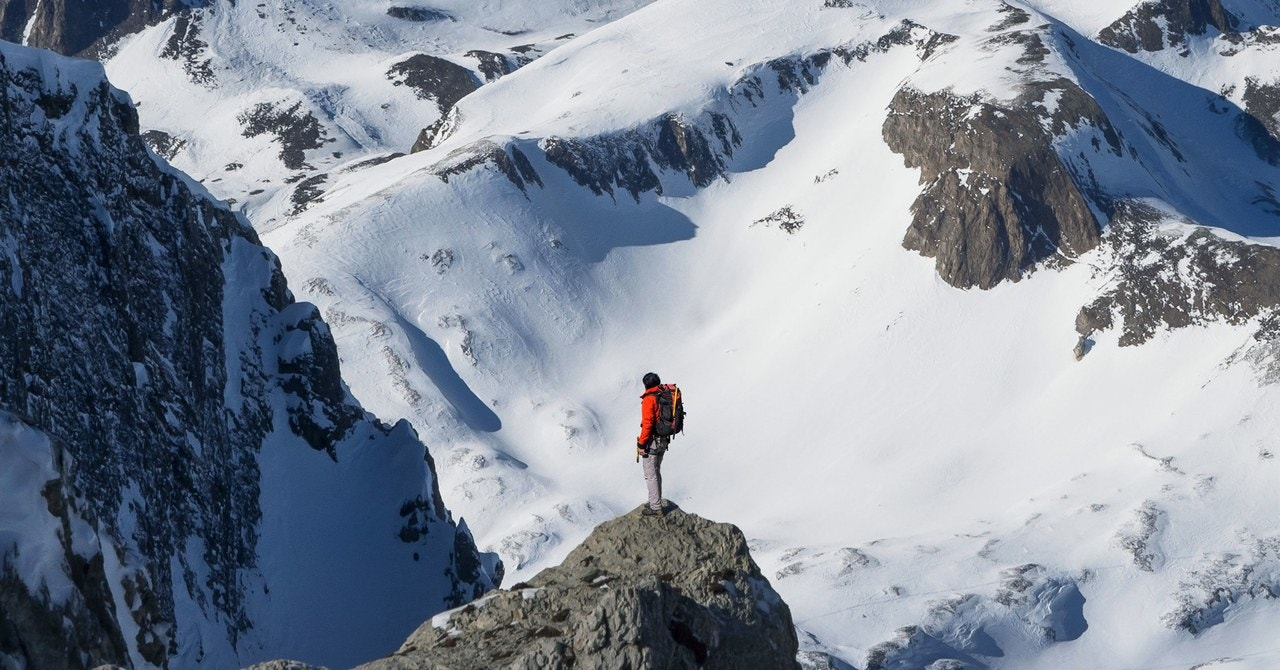# Wait, Is That Backpack … Floating?That’s all fine and everything until the human loses contact with the ground. At that point, there are only two forces on the human—the downward gravitational force and the downward spring force from the pack. That yields a downward acceleration greater than what it would be from gravity alone. At the same time, the pack is still being pushed up by the spring force. These two things together cause a vertical separation between the pack and the human.

OK, just to make sure everything sinks in, how about a quick homework question? What would happen if you used a backpack with a very low mass? Would this change the separation distance between the human and the pack? (You can use the code above to find the answer.)

How to Beat the Bounce

When we started you probably thought, “OK, the HoverGlide has a springlike shock absorber built into it—end of story.” But now we’ve seen that even a regular backpack has a kind of springlike connection in the harness. So the key isn’t having a spring per se, it’s having the right spring.

Specifically, HoverGlide uses a spring with a much lower spring constant k. Because of that, the pack takes a much longer time to accelerate upward, because the spring exerts a smaller force. By the time the human comes back down, the pack really hasn’t even moved. Since the pack barely moves, you don’t get that jarring impact when it collides with your shoulder.

Here’s a demonstration of this effect with a 1-kg mass hanging from a rubber band.

See how my hand is moving much more than the hanging weight? That’s the idea. This is actually a very interesting physics problem. Every introductory textbook looks at something similar but more boring—a mass oscillating on a spring. But what if the attachment point for the top of the spring is also oscillating? I call this the “jiggle spring.”

You know what’s super great about this problem? There’s two different ways to model it. The first uses Lagrangian mechanics to determine the motion by looking at the energy and the constraints on the system. That’s all I’m going to say about Lagrangian. It’s the best solution, but it would take a bit of explanation to get there.

Instead, I’m going to do what you know I like to do—a numerical calculation using Python. The main idea of a numerical calculation is to break the problem into very small steps in time. At each step, I make some simplifying assumptions to calculate the new position and momentum. (If you want a more detailed tutorial, here is how you would model an oscillating mass with a stable hanging point, not a jiggle spring.)